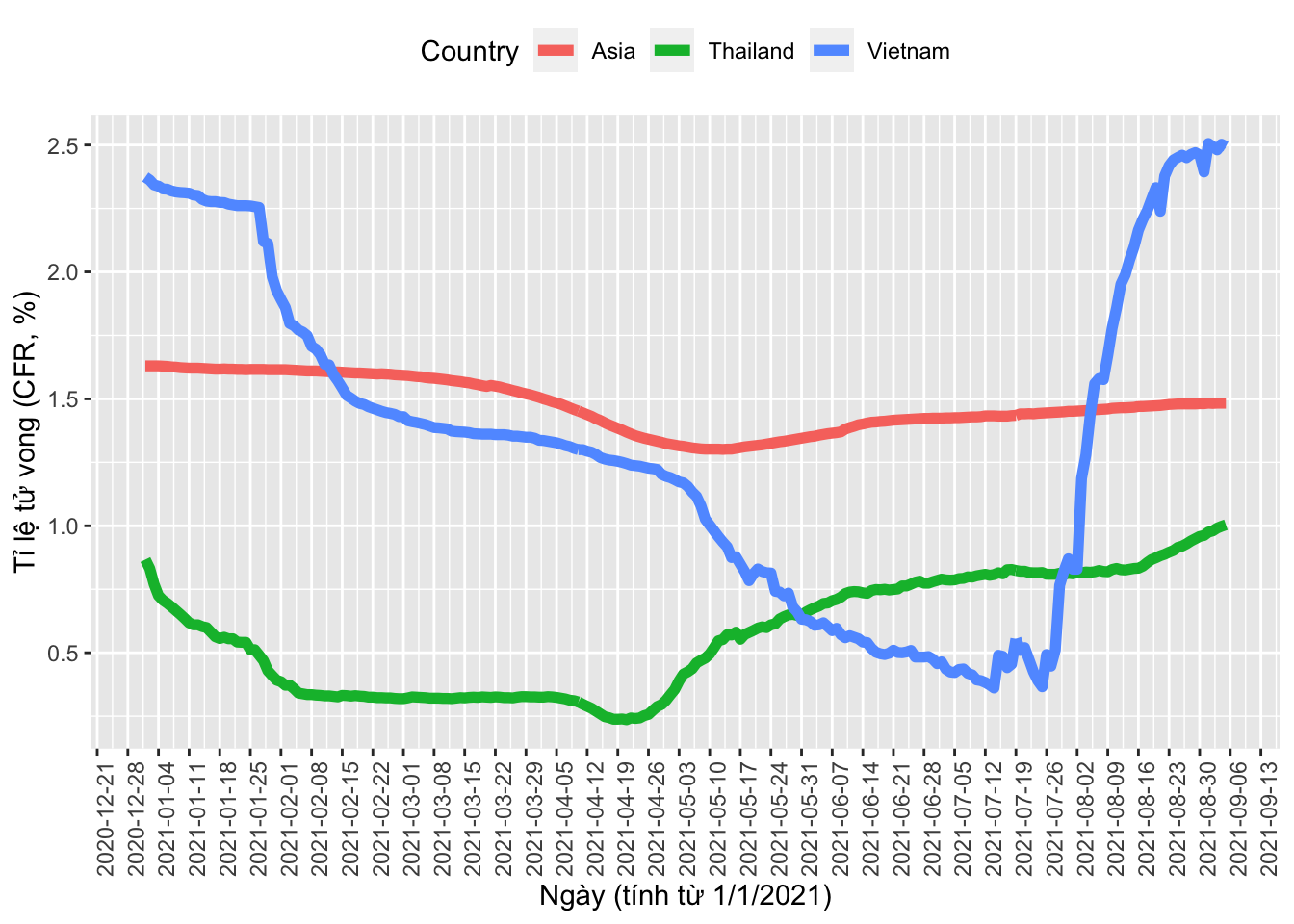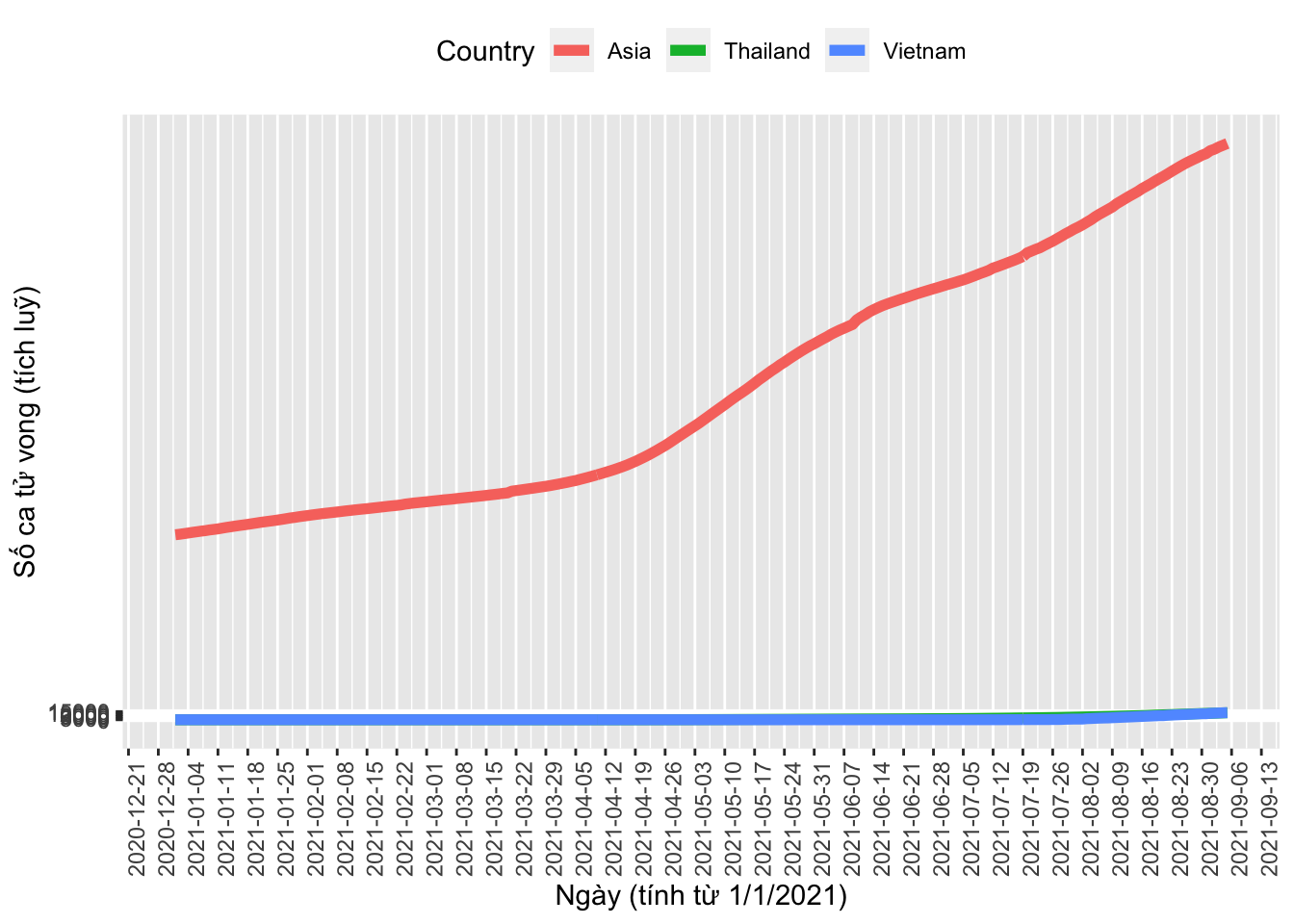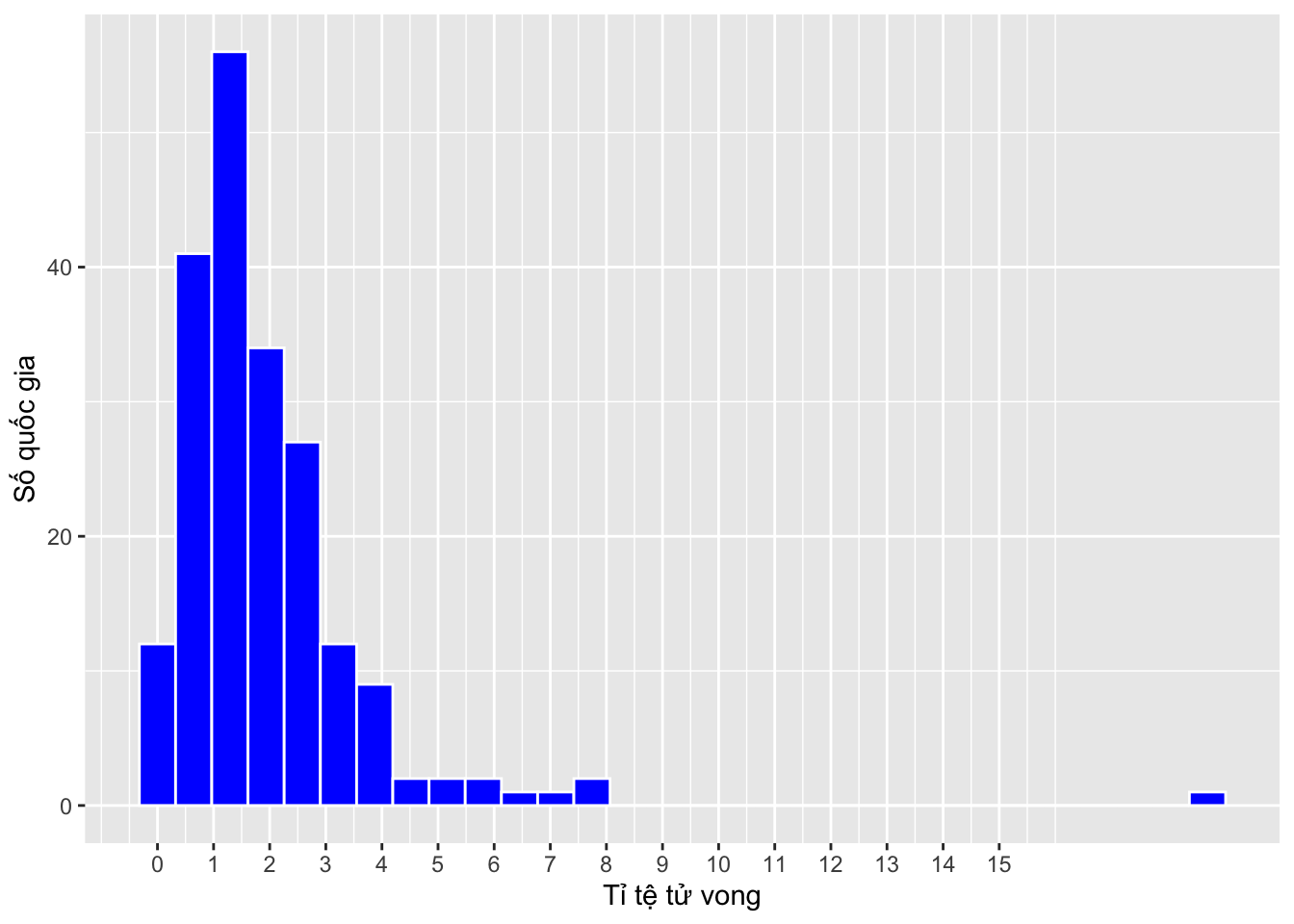## Warning: package 'ggplot2' was built under R version 4.0.2
library(ggrepel); library(tidyverse)
## Warning: package 'tidyverse' was built under R version 4.0.2
## ── Attaching packages ─────────────────────────────────────── tidyverse 1.3.1 ──
## ✓ tibble  3.1.2     ✓ dplyr   1.0.7
## ✓ tidyr   1.1.3     ✓ stringr 1.4.0
## ✓ readr   1.4.0     ✓ forcats 0.5.1
## ✓ purrr   0.3.4
## Warning: package 'tibble' was built under R version 4.0.2
## Warning: package 'tidyr' was built under R version 4.0.2
## Warning: package 'readr' was built under R version 4.0.2
## Warning: package 'dplyr' was built under R version 4.0.2
## Warning: package 'stringr' was built under R version 4.0.2
## Warning: package 'forcats' was built under R version 4.0.2
## ── Conflicts ────────────────────────────────────────── tidyverse_conflicts() ──

# From https://www.worldometers.info/coronavirus
dat2 = read_excel("~/Dropbox/Bao chi/Khoa hoc & Y te/Mortality analysis VN/Covid data 28-8-2021.xlsx")
dat2$CFR = dat2$Deaths/dat2$Cases*100 # From https://ourworldindata.org/grapher/deaths-covid-19-vs-case-fatality-rate df = read.csv("~/Dropbox/Bao chi/Khoa hoc & Y te/Mortality analysis VN/deaths-covid-19-vs-case-fatality-rate.csv") df$Date = as.Date(df\$Day, "%d/%m/%Y")
t1 = subset(df, Country=="Vietnam" | Country=="Thailand")
t2 = subset(df, Country=="Asia")
temp = rbind(t1, t2)
dat = filter(temp, Date>="2021-01-01")
ggplot(data=dat, aes(x=Date, y=CFR, col=Country)) + geom_line(lwd=2) + scale_x_date(date_breaks = "1 week") + theme(axis.text.x = element_text(angle = 90, hjust = 1)) + labs(x="Ngày (tính từ 1/1/2021)", y="Tỉ lệ tử vong (CFR, %)") + theme(legend.position="top")ggplot(data=dat, aes(x=Date, y=Deaths, col=Country)) + geom_line(lwd=2) + scale_x_date(date_breaks = "1 week") + scale_y_continuous(breaks = seq(from = 0, to = 15000, by = 3000)) + theme(axis.text.x = element_text(angle = 90, hjust = 1)) + labs(x="Ngày (tính từ 1/1/2021)", y="Số ca tử vong (tích luỹ)") + theme(legend.position="top")ggplot(data=dat2, aes(x=CFR)) + geom_histogram(color="white", fill="blue") + scale_x_continuous(breaks = seq(from = 0, to = 15, by = 1)) + labs(x="Tỉ tệ tử vong", y="Số quốc gia")
## stat_bin() using bins = 30. Pick better value with binwidth.